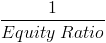# Equity Multiplier

Equity Multiplier Calculator (Click Here or Scroll Down)The formula for equity multiplier is total assets divided by stockholder's equity. Equity multiplier is a financial leverage ratio that evaluates a company's use of debt to purchase assets.

## Use of Equity Multiplier Formula

The equity multiplier formula is used in the return on equity DuPont formula for the financial leverage portion of DuPont analysis. Broadly speaking, financial leverage is used in financial analysis to evaluate a company's use of debt.

To understand how the equity multiplier formula is related to debt, it should be noted that in finance, a company's assets equal debt plus equity. Debt is not specifically referenced in the equity multiplier formula, but it is an underlying factor in that total assets in the numerator of the formula for the equity multiplier includes debt. This can be shown by restating total assets in the equity multiplier formula as debt plus equity.

## Alternative Equity Multiplier FormulaAn alternative formula for the equity multiplier is the reciprocal of the equity ratio. As previously stated, a company's assets are equal to debt plus equity. Therefore, the equity ratio calculates the equity portion of a company's assets. This ratio in the denominator of the formula can also be found by subtracting one minus the debt ratio.

New to Finance?